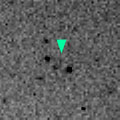# \$B%t%!%\$%5%i(B-\$B%*%F%k%^WB@1(B

139P/Vaisala-Oterma (1998)###\$B%W%m%U%#!<%k(B

 \$BId9f(B 139P/1998 WG22 \$B:FH/8+F|(B 1998\$BG/(B11\$B7n(B18\$BF|(B \$B:FH/8+8wEY(B 19.0\$BEy(B \$B:FH/8+ Lincoln Laboratory Near-Earth Asteroid Research project

###\$B###\$B50F;MWAG(B

```   The following improved orbital elements by Kenji Muraoka, are
from 86 observations 1939 to 1999, perturbations by 9 Plantes,
Moon and 5 minor planets were taken into account.
The mean residual is +/- 0.82 arc seconds.

Epoch  =  1998 Sept. 24.0  TT      JDT = 2451080.5
T  =  1998 Sept. 28.80925      +/- 0.04745 (m.e.) TT
Peri. =  165.54767                +/- 0.01960
Node  =  242.46830                +/- 0.01086   (2000.0)
Incl. =    2.33247                +/- 0.00036
q  =    3.3814917              +/- 0.0000700 AU
e  =    0.2483321              +/- 0.0000187
a  =    4.4986515              +/- 0.0000323 AU
n  =    0.10329530             +/- 0.00000111
P  =    9.542                  +/- 0.0001029  years
```

###\$B@1?^(B###\$B8wEYJQ2=(B

```        m1 = 11.0 + 5 log\$B&\$(B + 10.0 log r
```##### \$B50F;MWAG\$OB<2,7r<#;a\$N7W;;\$K\$h\$k\$b\$N\$G\$9!#(B \$B@1?^\$O(B StellaNavigator Ver.2.0 for Windows (\$B%"%9%H%m%"!<%D(B \$BJTCx(B / \$B%"%9%-!<=PHG6I4)(B) \$B\$G:n@.\$7\$?\$b\$N\$G\$9!#(B \$B8wEY%0%i%U\$O(BComet for Windows\$B\$G:n@.\$7\$?\$b\$N\$G\$9!#(B